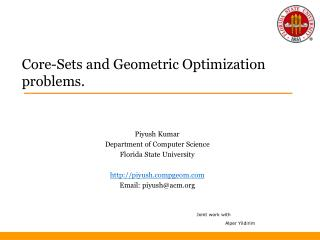# Core-Sets and Geometric Optimization problems. - PowerPoint PPT PresentationDownload PresentationCore-Sets and Geometric Optimization problems.

Core-Sets and Geometric Optimization problems.Download Presentation## Core-Sets and Geometric Optimization problems.

- - - - - - - - - - - - - - - - - - - - - - - - - - - E N D - - - - - - - - - - - - - - - - - - - - - - - - - - -
##### Presentation Transcript

1. Core-Sets and Geometric Optimization problems. Piyush Kumar Department of Computer Science Florida State University http://piyush.compgeom.com Email: piyush@acm.org Joint work with Alper Yildirim

2. Talk Outline • Introduction to Core-Sets for Geometric Optimization Problems. • Problems and Applications • Minimum Enclosing Balls (Next talk) • Axis Aligned Minimum Volume Ellipsoids • Motivation • Optimization Formulation/IVA • Algorithm • Computational Experiments • Future Directions.

3. r* Geometric Clustering • In order to cluster, we need • Points ( Polyhedra / Balls / Ellipsoids ? ) • A distance measure • Assume Euclidian unless otherwise stated. • A method for evaluating our clustering. • (We look at k-centers, 1-Ecenter, 1-center, • kernel 1-center, k-line-centers)

4. Fitting/Covering Problems • For some shapes, the problem is convex and hence tractable. (MEB / MVE / AAMVE). • Minimize the maximum distance • O(n) in 2D but becomes harder in higher dimensions. • Least squares / SVM Regression / … • d ≠ O(1)?

5. Fitting multiple subspaces/shapes? • Non-Convex (Min the max distance) / Non-Linear / NP-Hard to approx. • Has many applications • Minimize sum of distances (orthogonal) : SVD • Other assumptions : GPCA/PPCA/PCA…

6. Core-Sets Core Sets are a small subset of the input points whose fitting/covering is “almost” same as the fitting/covering of the entire input. [AHV06 Survey on Core-Sets]

7. Centers Core Set points Core-Sets

8. Core-Sets : Why Care?? • Because they are small ! • Hence we can work on large data sets • Because if we can solve the Optimization problem for Core Sets, we are guaranteed to be near the optimal for the entire input • Because they are easy to compute. • Most algorithms are practical. • Because they exist for even infinite point sets (MEB of balls , ellipsoids, etc)

9. Core-Sets Summary of known results for high dimensions.

10. High Level Algorithm (for most core-set algorithms) Compute an initial approximation of the solution and its core-set. 2. Find the furthest violator q. 3. Add q to the current core-set and update the corresponding solution. 4. Goto 2 if the solution is not good enough.

11. Motivation. • Optimization Formulation. • Initial Volume Approximation. • Algorithm. • Computational Experiments. Axis Aligned Minimum Volume Ellipsoids

12. Motivation • Collision Detection [Eberly 01] • Bounding volume Hierarchies • Machine Learning [BJKS 04] • Kernel clustering between MVEs and MEBs?

13. Optimization Formulation Volume of unit ball in n-dim space.

14. Optimization Formulation Convex

15. Optimization Formulation

16. High Level Algorithm (for most core-set algorithms) Compute an initial approximation of the solution and its core-set. 2. Find the furthest point q from the current solution. 3. Add q to the current core-set and update the corresponding ellipsoid. 4. Goto 2 if the solution is not good enough.

17. Optimization Formulation: Lemma 1

18. Initial Volume Approximation Output :

19. Feasible solution of (LD) Furthest point from current ellipsoid. Quality measure of current ellipsoid.

20. Feasible solution of (LD) Furthest point from current ellipsoid. Quality measure of current ellipsoid.

21. Increase weight for furthest point while decreasing it for remaining Points ensuring feasibility for (LD)

22. What the algorithm outputs? Complexity:

23. Computational Experiments Implementation in Matlab

24. MVE/AAMVE with outliers • k-AAMVE Coverings. • Distribution dependent tighter • core-set bounds? • Better practical methods? Future Work Acknowledgements NSF CAREER for support.